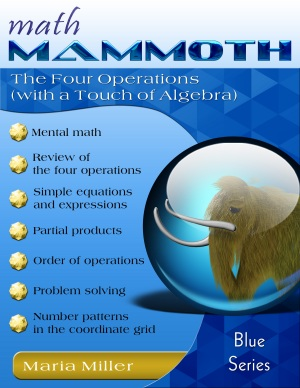^
You are here: HomeBlue Series → The Four Operations

# Math Mammoth The Four Operations (with a Touch of Algebra)129 pages

## Prices & ordering

Printed copy \$11.25

Math Mammoth The Four Operations (with a Touch of Algebra) is a mathematics worktext meant primarily for fifth and sixth grades. Some of the lessons can also be used in seventh grade. A worktext means that the book is a textbook and workbook together: the lessons include both the explanations of the concepts, as well as practice exercises.

The PDF version of this book is enabled for annotation. This means that if you prefer, the student can fill it in on the computer, using the typewriter and drawing tools in Adobe Reader version 9 or greater.

The main topics studied in this book are simple equations, expressions that involve a variable, the order of operations, long multiplication, long division, and graphing simple linear functions. Students encounter the exact definition of an equation and an expression. They practice the order of operations with problems that also reinforce the idea of the equal sign (“=”) as denoting equality of the right and left sides of an equation. This kind of exercises is needed because children may think that an equal sign signifies the act of finding the answer to a problem (as in 134 + 23 = ?, for example), which is not so.

Students solve addition and subtraction equations both with the help of diagrams (a.k.a. bar models) and also without. These diagrams (the same kind as in Singapore Math) are also used for simple multiplication and division equations and for mixture equations, such as 4x + 38 = 128.

We also present lessons on multi-digit multiplication (multiplying in columns). These lessons go farther than just reviewing the well-known algorithm. We study in detail: multiplying in parts (partial products), how those partial products can be seen in the algorithm itself, and how multi-digit multiplication can be visualized geometrically. Students also practice long division, including two-digit divisors, as a review from fourth grade.

Although the book is named, “The Four Operations,” please notice that the idea is not to practice each of the four operations separately, but rather to see how they are used together in solving problems and in simple equations. We are trying to develop student's algebraic thinking, including the abilities to: translate problems into mathematical operations, comprehend the many operations needed to yield an answer to a problem, “undo” operations, and so on. Many of the ideas in this chapter are preparing them in advance for algebra.

This book is suitable for 5th and 6th grades. It includes both the teaching material (lessons) as well as the exercises in the same book. The answer key is appended.

You might also be interested in:

## Better Yet - Bundle Deals!

*BONUS*: Buy any bundle listed above, and get the fully upgraded Soft-Pak software for FREE (includes 4 math and 2 language arts programs, complete with on screen and printable options).

## How and where to order

You can buy Math Mammoth books at:

• Here at MathMammoth.com website — simply use the "Add to cart" buttons you see on the product pages.
• Rainbow Resource carries printed copies for the Light Blue series books, plus several CDs (Light Blue and Blue series).
• At Teachers Pay Teachers you can purchase the Light Blue Series downloads, plus topical units.
• Lulu sells printed copies for most of the Math Mammoth materials (various series).

By purchasing any of the books, permission IS granted for the teacher (or parent) to reproduce this material to be used with his/her students in a teaching situation; not for commercial resale. However, you are not permitted to share the material with another teacher.

In other words, you are permitted to make copies for the students/children you are teaching, but not for other teachers' usage.

Math Mammoth books are PDF files. You will need Adobe Reader to view them, including if you use a Mac or Linux. You can try other PDF viewers, but they seem to either omit or mess up some of the images.
WAIT!

Receive my monthly collection of math tips & resources directly in your inbox — and get a FREE Math Mammoth book!You can unsubscribe at any time.

### Math Mammoth Tour

Confused about the different options? Take a virtual email tour around Math Mammoth! You'll receive:

An initial email to download your GIFT of over 400 free worksheets and sample pages from my books. Six other "TOURSTOP" emails that explain the important things and commonly asked questions concerning Math Mammoth curriculum. (Find out the differences between all these different-colored series!)

This way, you'll have time to digest the information over one or two weeks, plus an opportunity to ask me personally about the curriculum.
A monthly collection of math teaching tips & Math Mammoth updates (unsubscribe any time)### "Mini" Math Teaching Course

This is a little "virtual" 2-week course, where you will receive emails on important topics on teaching math, including:

- How to help a student who is behind
- Troubles with word problems
- Teaching multiplication tables
- Why fractions are so difficult
- The value of mistakes
- Should you use timed tests
- And more!

A GIFT of over 400 free worksheets and sample pages from my books right in the very beginning.A monthly collection of math teaching tips & Math Mammoth updates (unsubscribe any time)
Enter your email to receive math teaching tips, resources, Math Mammoth news & sales, humor, and more! I tend to send out these tips about once monthly, near the beginning of the month, but occasionally you may hear from me twice per month (and sometimes less often).• A GIFT of over 400 free worksheets and sample pages from my books.